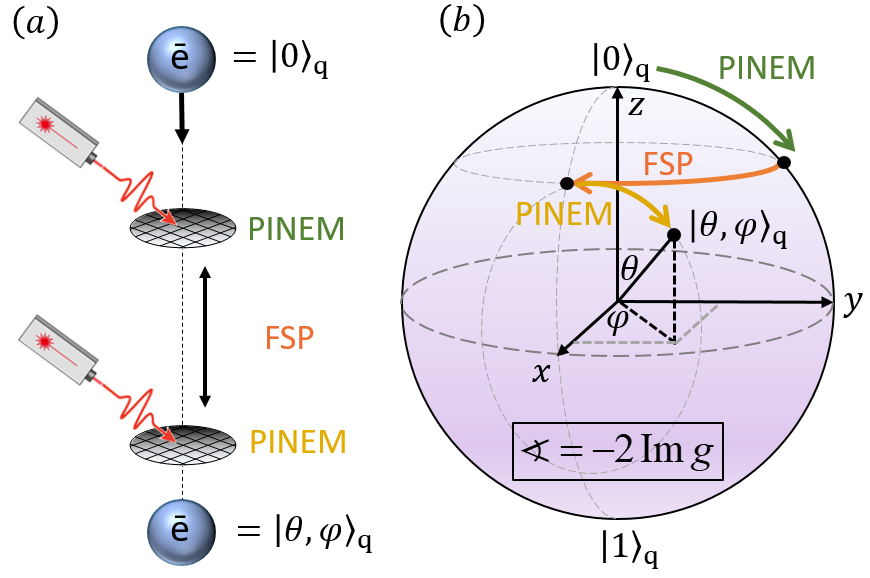# Free Electron Qubits

Free-electron interactions with laser-driven nanophotonic nearfields can quantize the electrons’ energy spectrum and provide control over this quantized degree of freedom. We propose to use such interactions to promote free electrons as carriers of quantum information and find how to create a qubit on a free electron, holding promise for applications in electron microscopy and spectroscopy. We find how to implement the qubit’s non-commutative spin-algebra, then control and measure the qubit state with a universal set of 1-qubit gates. These gates are within the current capabilities of femtosecond-pulsed laser-driven transmission electron microscopy. Pulsed laser driving promise configurability by the laser intensity, polarizability, pulse duration, and arrival times.The quantum gates: Controlling a free-electron qubit. (a) Illustration of an experimental scheme implementing a quantum gate on a free-electron qubit. A pre-designed quantum computation is translated into gates, mapped to PINEMs and FSPs, and implemented inside the UTEM. (b) Geometrical representation of the electron qubit and gates on a Blochsphere corresponding to the implementation in (a), showing the transformation from the $$\left| 0 \right\rangle$$ state to an arbitrary point on the Bloch sphere. PINEM operations serve as continuous rotations around the $$x$$ -axis, and FSP operations as $$\pi / 2$$ rotations around the $$z$$ -axis, which together form a universal set of 1-qubit gates.

Back